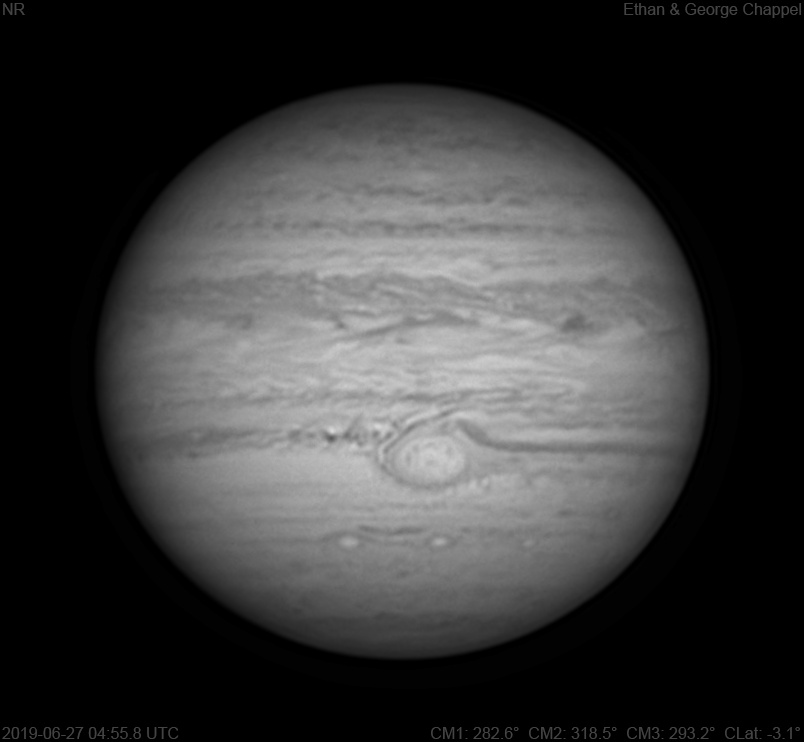# Jupiter 2019-06-27 04:55 UTC

CM1: 282.60°

CM2: 318.50°

CM3: 293.20°

CLat: -3.10°

Description

Seeing seemed to improve a bit, which helped the near-infrared filter that already cuts through seeing better than RGB filters.

Notes:

• White Oval Z is east of the central meridian.
• The Great Red Spot is at the central meridian.
• A small storm is on the northern edge of the darkened GRS hollow.
• The northern edge of the GRS appears to be touching the small storm embedded in the GRS hollow, which is not the case in the RGB image.
• Dark clouds from the GRS hollow appear to be entering the South Tropical Zone, with a wavy pattern on the southern edge.

Equipment

ZWO ASI290MM

Celestron EdgeHD 14

Logs
```FireCapture v2.6  Settings
------------------------------------
Observer=Ethan Chappel
Camera=ZWO ASI290MM
Filter=IR
Profile=Jupiter
Diameter=45.61"
Magnitude=-2.59
CMI=282.0° CMII=317.9° CMIII=292.6°  (during mid of capture)
FocalLength=7800mm (F/27)
Resolution=0.08"
Filename=2019-06-27-0454_8-EC-IR-Jup.ser
Date=2019_06_27
Start=04_53_49.344
Mid=04_54_49.355
End=04_55_49.366
Start(UT)=04_53_49.344
Mid(UT)=04_54_49.355
End(UT)=04_55_49.366
Duration=120.022s
Date_format=yyyy_MM_dd
Time_format=HH_mm_ss
LT=UT -6h
Frames captured=7998
File type=SER
Binning=no
Bit depth=8bit
Debayer=no
ROI=812x698
ROI(Offset)=0x0
FPS (avg.)=66
Shutter=15.00ms
Gain=330 (55%)
AutoGain=off
USBTraffic=40 (off)
FPS=100 (off)
Gamma=50
SoftwareGain=10 (off)
AutoExposure=off
HighSpeed=off
Brightness=1 (off)
AutoHisto=75 (off)
Histogramm(min)=0
Histogramm(max)=151
Histogramm=59%
Noise(avg.deviation)=1.98
AutoAlign=false
PreFilter=none
Limit=120 Seconds
Sensor temperature=34.5°C
Focuser position=4845
FireCapture v2.6  Settings
------------------------------------
Observer=Ethan Chappel
Camera=ZWO ASI290MM
Filter=IR
Profile=Jupiter
Diameter=45.61"
Magnitude=-2.59
CMI=283.2° CMII=319.1° CMIII=293.8°  (during mid of capture)
FocalLength=7800mm (F/27)
Resolution=0.08"
Filename=2019-06-27-0456_9-EC-IR-Jup.ser
Date=2019_06_27
Start=04_55_54.391
Mid=04_56_54.397
End=04_57_54.404
Start(UT)=04_55_54.391
Mid(UT)=04_56_54.397
End(UT)=04_57_54.404
Duration=120.013s
Date_format=yyyy_MM_dd
Time_format=HH_mm_ss
LT=UT -6h
Frames captured=7998
File type=SER
Binning=no
Bit depth=8bit
Debayer=no
ROI=812x698
ROI(Offset)=0x0
FPS (avg.)=66
Shutter=15.00ms
Gain=330 (55%)
AutoGain=off
USBTraffic=40 (off)
FPS=100 (off)
Gamma=50
SoftwareGain=10 (off)
AutoExposure=off
HighSpeed=off
Brightness=1 (off)
AutoHisto=75 (off)
Histogramm(min)=0
Histogramm(max)=142
Histogramm=55%
Noise(avg.deviation)=1.94
AutoAlign=false
PreFilter=none
Limit=120 Seconds
Sensor temperature=34.5°C
Focuser position=4845
```